GMAT Math : Arithmetic

Example Questions

Example Question #3177 : Gmat Quantitative Reasoning

One of the managers in a certain company is to be selected at random to represent the company at a national conference. What is the probability that a woman will be selected to represent the company?

(1) One third of the managers in the company are women.

(2) Half of the employees in the company are women.

Statement (2) ALONE is sufficient, but Statement (1) ALONE is not sufficient.

Each Statement ALONE is sufficient.

Statements (1) and (2) TOGETHER are not sufficient.

Both statements TOGETHER are sufficient, but NEITHER statement ALONE is sufficient.

Statement (1) ALONE is sufficient, but Statement (2) ALONE is not sufficient.

Statement (1) ALONE is sufficient, but Statement (2) ALONE is not sufficient.

Explanation:

(1) One third of the managers in the company are women.

Therefore the probability that the manager selected at random is a woman is.

Statement (1) alone is sufficient.

(2) Half of the employees in the company are women.

Even though half of the company's employees are women, this statement doesn't give the proportion of women who hold a manager job. Therefore, Statement (2) is not sufficient.

Example Question #3178 : Gmat Quantitative Reasoning

What is the probability that a randomly selected employee at a company have a MBA degree?

(1)Employees have a MBA degree.

(2)percent of employees do not have a MBA degree.

Both statements TOGETHER are sufficient, but NEITHER statement ALONE is sufficient.

Statements (1) and (2) TOGETHER are not sufficient.

Statement (1) ALONE is sufficient, but Statement (2) ALONE is not sufficient.

Each Statement ALONE is sufficient.

Statement (2) ALONE is sufficient, but Statement (1) ALONE is not sufficient.

Statement (2) ALONE is sufficient, but Statement (1) ALONE is not sufficient.

Explanation:

(1) 150 Employees have a MBA degree.

Since we do not know the number of employees at the company, we cannot determine the probability of employees who have an MBA degree. Statement (1) is not sufficient.

(2) 70 percent of employees do not have a MBA degree.

We can then determine the percentage of employees with an MBA degree as 30 percent. Therefore the probability of employees who have an MBA degree is 0.3.

Statement (2) ALONE is sufficient.

Example Question #3179 : Gmat Quantitative Reasoning

An insurer is trying to determine the probability of policyholders who had an accident this year.

(1) Of all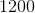policyholders,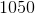did not have an accident.

(2) Of the policyholders who had an accident,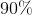received a claim payment.

Each Statement ALONE is sufficient.

Statement (2) ALONE is sufficient, but Statement (1) ALONE is not sufficient.

Statement (1) ALONE is sufficient, but Statement (2) ALONE is not sufficient.

Statements (1) and (2) TOGETHER are not sufficient.

Both statements TOGETHER are sufficient, but NEITHER statement ALONE is sufficient.

Statement (1) ALONE is sufficient, but Statement (2) ALONE is not sufficient.

Explanation:

(1) Of all 1200 policyholders, 1050 did not have an accident.

From statement (1), we can determine that 150 policyholders had an accident. We can then calculate the probability as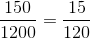.

Statement (1) alone is sufficient.

(2) Of the policyholders who had an accident, 90% received a claim payment.

Statement (2) is irrelevant since we are not interested in the probability of policyholders who received a claim payment.

Statement (2) is not sufficient.

Example Question #3180 : Gmat Quantitative Reasoning

A store owner is trying to determine the probability of defective light bulbs he holds in his inventory.

(1)out oflight bulbs are defective.

(2) After checking half of the inventory, the store owner findsdefective light bulbs.

Each Statement ALONE is sufficient.

Statements (1) and (2) TOGETHER are not sufficient.

Statement (2) ALONE is sufficient, but Statement (1) ALONE is not sufficient.

Statement (1) ALONE is sufficient, but Statement (2) ALONE is not sufficient.

Both statements TOGETHER are sufficient, but NEITHER statement ALONE is sufficient.

Statement (1) ALONE is sufficient, but Statement (2) ALONE is not sufficient.

Explanation:

(1) 15 out of 150 light bulbs are defective.

Using statement (1), the probability of defective light bulbs is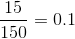Statement (1) is sufficient.

(2) After checking half of the inventory, the store owner finds 10 defective light bulbs

We do not know the number of defective light bulbs in all the inventory and we do not know the number of light bulbs in inventory.

Statement (2) is not sufficient.

Example Question #3181 : Gmat Quantitative Reasoning

What is the number of unemployed adults in City A?

(1) The number of adults in City A is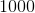.

(2) Three fourth of adults in City A are employed.

Statement (1) ALONE is sufficient, but Statement (2) ALONE is not sufficient.

Statement (2) ALONE is sufficient, but Statement (1) ALONE is not sufficient.

Statements (1) and (2) TOGETHER are not sufficient.

Both statements TOGETHER are sufficient, but NEITHER statement ALONE is sufficient.

Each Statement ALONE is sufficient.

Both statements TOGETHER are sufficient, but NEITHER statement ALONE is sufficient.

Explanation:

(1) The number of adults in City A is 1000.

Statement (1) is not sufficient to find the number of unemployed adults in City A.

(2) Three fourth of adults in City A are employed.

This statement tell us thatof adults are unemployed. However, without knowing the number of adults in City A we cannot determine the number of unemployed adults in City A. Statement (2) alone is not sufficient.

Combining both statements, we find that the number of unemployed adults isof 1000 which is 250.

Both statements together are sufficient, but neither statement alone is sufficient.

Example Question #3182 : Gmat Quantitative Reasoning

What is the probability that a randomly selected man in a sample of men has a beard?

(1) Half of the men in the sample do not have a beard.

(2) There aremen with a beard in the sample.

Both statements TOGETHER are sufficient, but NEITHER statement ALONE is sufficient.

Statement (1) ALONE is sufficient, but Statement (2) ALONE is not sufficient.

Statement (2) ALONE is sufficient, but Statement (1) ALONE is not sufficient.

Statements (1) and (2) TOGETHER are not sufficient.

Each Statement ALONE is sufficient.

Statement (1) ALONE is sufficient, but Statement (2) ALONE is not sufficient.

Explanation:

(1) Half of the men in the sample do not have a beard.

This statement implies that the other half of the men in the sample have a beard. Therefore, the probability that a randomly selected man in the sample has a beard is 0.5.

Statement (1) alone is sufficient.

(2) There are 100 men with a beard in the sample.

Statement (2) gives us the number of men who have a beard in the sample but without knowing the number of men in the sample, we cannot find the probability of men who have a beard.

Statement (2) is not sufficient.

Example Question #3183 : Gmat Quantitative Reasoning

What is the probability that a randomly selected student enrolled at a university will be a graduate student?

(1)of the students enrolled at the university are graduate students.

(2) Onlyof the students enrolled are graduate students.

Statements (1) and (2) TOGETHER are not sufficient.

Each Statement ALONE is sufficient.

Statement (1) ALONE is sufficient, but Statement (2) ALONE is not sufficient.

Statement (2) ALONE is sufficient, but Statement (1) ALONE is not sufficient.

Both statements TOGETHER are sufficient, but NEITHER statement ALONE is sufficient.

Statement (2) ALONE is sufficient, but Statement (1) ALONE is not sufficient.

Explanation:

(1) 1000 of the students at the university are graduate students.

Statement (1) alone is not sufficient because we don't know the total number of students enrolled at the university.

(2) Only 20% of the students enrolled are graduate students.

Statement (2) gives the probability of graduate students enrolled at that university as 20%(0.2).

Statement (2) Alone is sufficient.

Example Question #3184 : Gmat Quantitative Reasoning

A bag contains red, yellow and green marbles. There are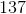marbles total.

I) There are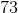green marbles.

II) The number of yellow marbles is half of one less than the number of green marbles.

What are the odds of picking a red followed by a green followed by a yellow? Assume no replacement.

Statement I is sufficient to answer the question, but statement II is not sufficient to answer the question.

Either statement is sufficient to answer the question.

Statement II is sufficient to answer the question, but statement I is not sufficient to answer the question.

Both statements are needed to answer the question.

Both statements are needed to answer the question.

Explanation:

In order to calculate the probability the question asks for, we need to know the number of each color of marble.

I) Gives us the number of greens.

II) Gives us clues which will allow us to find the number of reds and yellows.

We need both statements to answer this question.

Example Question #3185 : Gmat Quantitative Reasoning

In a standard deck of cards (4 suits, 13 cards of each suit) 3 cards have been removed. What are the odds of choosing one red card followed by 2 black cards? Assume replacement.

I) Two of the cards that were removed were black.

II) One of the removed cards was red.

Either statement is sufficient to answer the question.

Statement I is sufficient to answer the question, but statement II is not sufficient to answer the question.

Statement II is sufficient to answer the question, but statement I is not sufficient to answer the question.

Both statements are needed to answer the question.

Either statement is sufficient to answer the question.

Explanation:

To find the desired probability we need to know which color cards were removed.

I) Tells us the number of black cards removed.

II) Tells us the number of red cards removed.

At first glance, we need both statements, but if we think for a minute, if we know the total number of cards removed and the number of one color removed, we can find the number of the color... So either statement works.

Example Question #3186 : Gmat Quantitative Reasoning

Holly took nine cards out of a standard 52-card deck. Did she take out more red cards than black, or vice versa?

Statement 1: Holly ran 100 experiments using the modified deck, each involving a shuffle and a draw; she drew a red card 45 times.

Statement 2: Susan ran 100 experiments using the modified deck, each involving a shuffle and a draw; she drew a red card 44 times.

BOTH statements TOGETHER are sufficient to answer the question, but NEITHER statement ALONE is sufficient to answer the question.

Statement 1 ALONE is sufficient to answer the question, but Statement 2 ALONE is NOT sufficient to answer the question.

EITHER statement ALONE is sufficient to answer the question.

BOTH statements TOGETHER are insufficient to answer the question.

Statement 2 ALONE is sufficient to answer the question, but Statement 1 ALONE is NOT sufficient to answer the question.